## Consider a circle whose equation is x2 + y2 – 2x – 8 = 0. Which statements are true? Select three options. The radiu

Question

Consider a circle whose equation is x2 + y2 – 2x – 8 = 0. Which statements are true? Select three options.

The radius of the circle is 3 units.

The center of the circle lies on the x-axis.

The center of the circle lies on the y-axis.

The standard form of the equation is (x – 1)² + y² = 3.

The radius of this circle is the same as the radius of the circle whose equation is x² + y² = 9.

in progress 0
6 months 2021-07-31T03:53:34+00:00 2 Answers 117 views 0

## Answers ( )

The first, second, and fifth statements are correct.

Step-by-step explanation:

i got it right on edg if right mark as brainliest

The first, second, and fifth statements are correct.

Step-by-step explanation:

We are given a circle with the equation: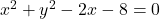And we want to select the statements that are true.

First, we can convert the equation into standard form. We can group each variable: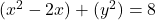And complete the square for the first term: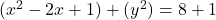Factor and simplify: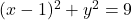We can rewrite our equation as: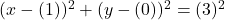So, this tells us that we have a circle centered on (1, 0) with a radius of 3 units.

In this case, the first statement, second statement (the point (1,0) is on the x-axis), and fifth statements are correct (the square root of 9 is also 3).